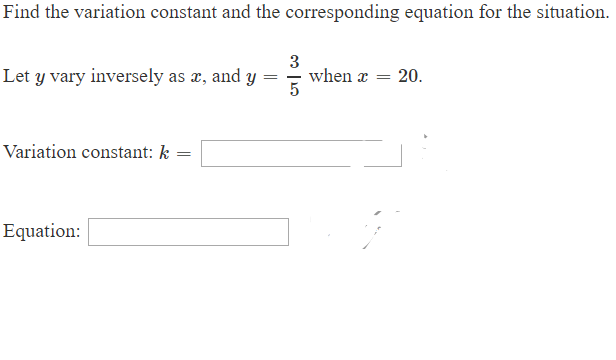# Find the variation constant and the corresponding equation for the situation when 20. 5 Let y vary inversely as a, and y _ Variation constant: k = Equation:

Questionhelp_outlineImage TranscriptioncloseFind the variation constant and the corresponding equation for the situation when 20. 5 Let y vary inversely as a, and y _ Variation constant: k = Equation: fullscreen

### Want to see this answer and more?

Experts are waiting 24/7 to provide step-by-step solutions in as fast as 30 minutes!*